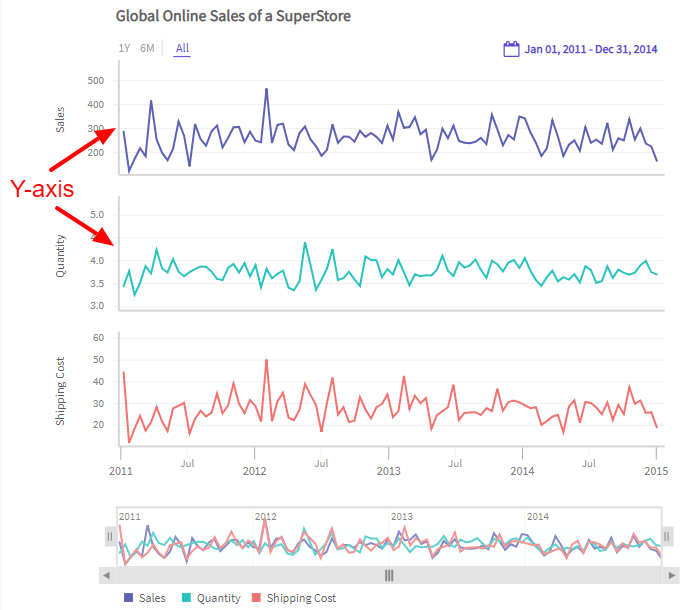# Y-Axis

A timeseries chart can have one or more Y-axes. The latter situation occurs when you need to build a multi-series or multivariate chart.

A Y-axis in a timeseries chart looks as shown in the image below:### Configure Y-axis

Use the `YAxes` object of the `timeSeries` instance of the `TimeSeriesChart` class to define a Y-axis. After that, use the following to configure the axes.

• Set the aggregation function using the `Aggregation` property of `YAxes` object. which accepts enum of type `TimeSeriesAggregation.Function`. `TimeSeriesAggregation.Function` can accept one of the enum values from `SUM`, `COUNT`, `MAX`, `MIN`, `LAST`, `FIRST`, `AVERAGE`, `VARIANCE` or `STDDEV`.

• Set the axis type using the `AxisType` property of the `YAxes` object, which accepts enum of type `TimeSeriesYAxisObject.YAxisType`. `TimeSeriesYAxisObject.YAxisType` can accept the following enum values:

• `LINEAR`
• `LOG`
• Set a prefix for the values on the Y-axis using the `Prefix` property of the `Format` object. `Prefix` accepts a string type parameter.

• Set a suffix for the values on the Y-axis using the `Suffix` property of the `Format` object. `Suffix` accepts a string type parameter.

• Set the maximum value for the Y-axis using the double type `Max` property of the `YAxes` object.

• Set the minimum value for the Y-axis using the double type `Min` property of the `YAxes` object.

• Set the plot type using the `PlotType` property of the `YAxes` object, which accepts enum of type `TimeSeriesYAxisObject.SeriesPlotType`. `TimeSeriesYAxisObject.SeriesPlotType` can accept the enum values from `LINE`, `COLUMN`, `AREA`, `SPLINE`, `STEPLINE`, `OHLC` and `CANDLESTICK`.

• Set the Y-axis title using the string type `Title` property of the `YAxes` object.

• Finally, to set the plot type for the chart, use the `Add()` method of the `Plot` object.

Refer to the code given below:

``````/* add different configuration */
timeSeries.YAxes.Aggregation = TimeSeriesAggregation.Function.COUNT;
timeSeries.YAxes.AxisType = TimeSeriesYAxisObject.YAxisType.LINEAR;
timeSeries.YAxes.Format.Prefix = "\$";
timeSeries.YAxes.Format.Suffix = "K";
timeSeries.YAxes.Max = 30000;
timeSeries.YAxes.Min = 10000;
timeSeries.YAxes.PlotType = TimeSeriesYAxisObject.SeriesPlotType.AREA;
timeSeries.YAxes.Title = "Total Sales";